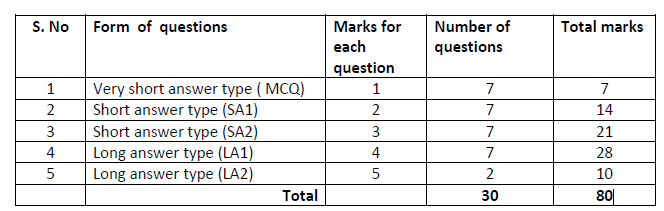# Goa Board Class 12 HSSC Maths Blueprint & Marks Weightage

Students can refer to the Goa Board Class 12 HSSC Maths Question Paper Blueprint & Marks Weightage to get an idea about the weightage of the distribution of marks over different dimensions of the question paper. As per the design of the model question paper-2018, a weightage of 18 marks is given from the total of 80 marks to the unit “Integrals and Applications”. Also, 12 marks weightage given to the unit “Vectors and 3D.” At the same time, about 45% of the weightage is given to the learning outcome “ understanding”.

## Goa Board Class 12 HSSC Maths Question Paper Marks Weightage and Blueprint PDFs

To get a complete overview of the marks weightage students can download the GBSHSE Class 12 HSSC Maths Exam Paper Blueprint & Marks Weightage from the links below. Students can refer to these Goa Board Class 12 HSSC Maths Question Paper Marks Weightage and Blueprint and plan their studies for the board exams.

## Goa Board HSSC Class 12 Maths Exam Question Paper Design PDF Download

### Weightage to Content/Subject units:

 Serial No. Units Marks 1. Relations and Functions 4 2. Inverse Trigonometric Functions 4 3. Continuity and Derivatives 10 4. Applications of Derivatives 6 5. Integrals and applications 18 6. Differential Equations 6 7. Vectors and 3D 12 8. Probability 6 9. Matrices 6 10. Determinants 4 11. Linear Programming 4 Total 80

### Weightage to Forms of QuestionsStudents can also get to know the unit-wise time and marks distribution for the exam paper from this blueprints and question paper designs given, based on which they can prepare most effectively for the exams to score high marks.

Get more details about Goa Board HSSC Class 12 Previous Year Question Paper and other study materials of GBSHSE from BYJU’S.